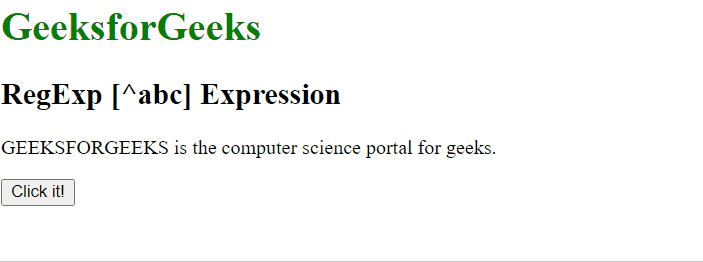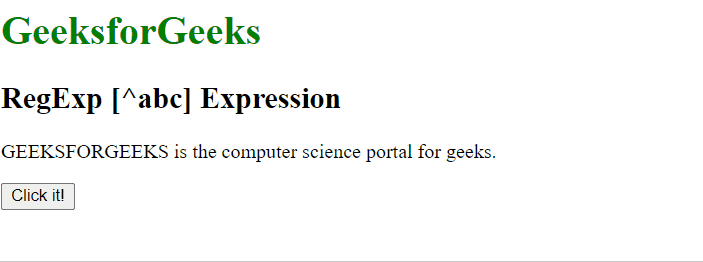Open in App
Not now

# JavaScript RegExp [^abc] Expression

• Last Updated : 06 Jan, 2023

The RegExp [^abc] Expression in JavaScript is used to search for any character which is not between the brackets. The character inside the brackets can be a single character or a span of characters.

• [A-Z]: It is used to match any character from uppercase A to uppercase Z.
• [a-z]: It is used to match any character from lowercase a to lowercase z.
• [A-z]: It is used to match any character from uppercase A to lowercase z.
• [abc…]: It is used to match any character between the brackets.

Syntax:

`/[^abc]/ `

or

`new RegExp("[^abc]")`

Syntax with modifiers:

`/\[^abc]/g `

or

`new RegExp("[^abc]", "g")`

Example 1: This example searches the characters which are not present between [A-G] i.e uppercase A to uppercase G in the whole string.

## html

 `<``h1` `style``=``"color:green"``>``    ``GeeksforGeeks```` ` `<``h2``>RegExp [^abc] Expression`` ` `<``p``>``    ``GEEKSFORGEEKS is the computer``    ``science portal for geeks.```` ` `<``button` `onclick``=``"geek()"``>``    ``Click it!```` ` `<``p` `id``=``"app"``>`` ` `<``script``>``    ``function geek() {``        ``var str1 = "GEEKSFORGEEKS is the computer "``                    ``+ "science portal for geeks.";``         ` `        ``var regex4 = /[^A-Z]/g;``        ``var match4 = str1.match(regex4);``     ` `        ``document.getElementById("app").innerHTML =``                ``"Found " + match4.length``                ``+ " matches: " + match4;``    ``}```

Output:RegExp [^abc] Expression

Example 2: This example searches the characters which are not present between [a-g] i.e lowercase a to lowercase g in the whole string.

## html

 `<``h1` `style``=``"color:green"``>``    ``GeeksforGeeks```` ` `<``h2``>RegExp [^abc] Expression`` ` `<``p``>``    ``GEEKSFORGEEKS is the computer``    ``science portal for geeks.```` ` `<``button` `onclick``=``"geek()"``>``    ``Click it!```` ` `<``p` `id``=``"app"``>`` ` `<``script``>``    ``function geek() {``        ``var str1 = "GEEKSFORGEEKS is the computer "``                    ``+ "science portal for geeks.";``         ` `        ``var regex4 = /[^a-p]/g;``        ``var match4 = str1.match(regex4);``     ` `        ``document.getElementById("app").innerHTML =``                    ``"Found " + match4.length``                    ``+ " matches: " + match4;``    ``}```

Output:RegExp [^abc] Expression

Supported Browsers: The browsers supported by RegExp [^abc] Expression are listed below: